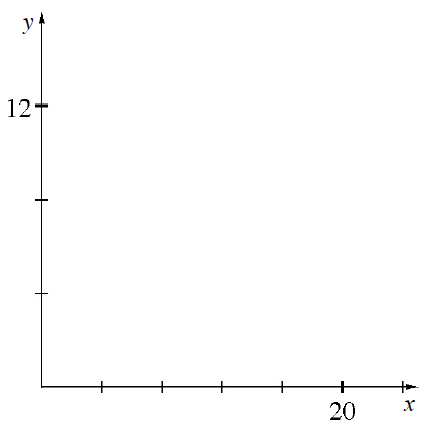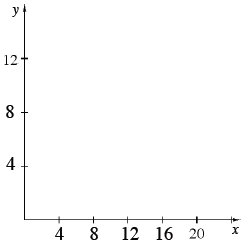### Home > CC2 > Chapter 2 > Lesson 2.3.1 > Problem2-115

2-115.

Complete the scaling for each number line or set of axes.

1.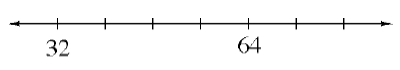Determine the size of each interval.

$\frac{64-32}{4}=\frac{32}{4}=8$

Add to or subtract from the numbers on the given points in order to fill in the missing numbers.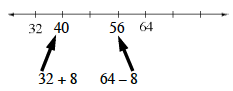1.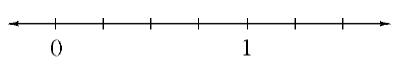Follow the steps given for part (a).

The intervals have a width of $0.25$ or $\frac{1}{4}$.

1.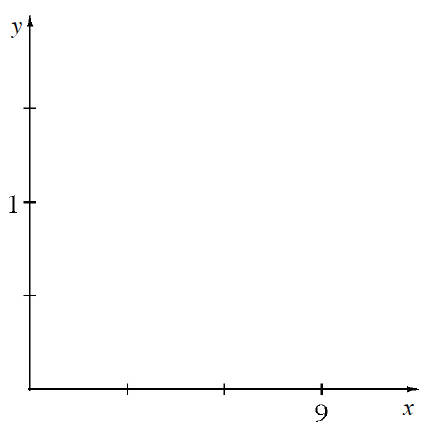Remember that the point where the axes intersect is $0$ on both axes.

Fill in each axis as you did the two number lines above. Remember that the two axes may have different scaling.

1.